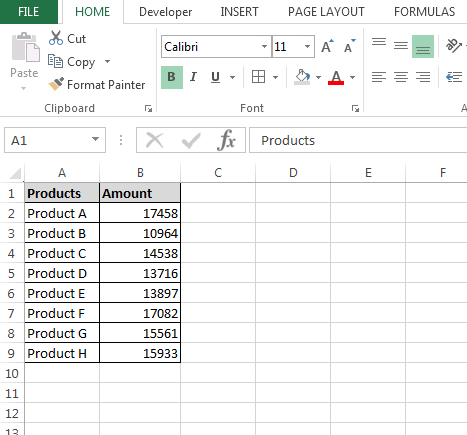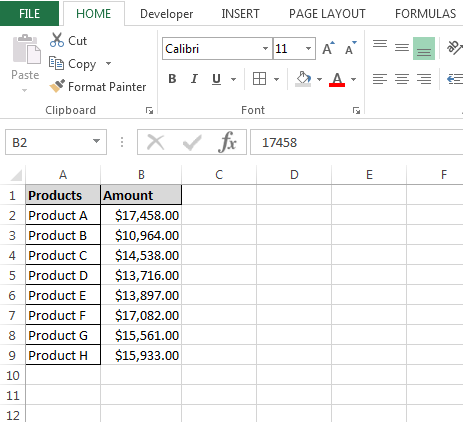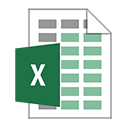How to Format Cells as Currency and where we can Use it

Let’s take an example to understand how and where we can format the cell in currency.

We have product’s amount data in range A1:B9 in which column A contains Product name and column B contains amount of it. We want to format the amount range as currency so that, for user, it should be easy to understand that a number is a currency.To write the macro, follow below given steps:-

• Open VBA Page and press the key Alt+F11.
• Insert a module.
• Write the below mentioned code:

Sub FormatCurrency()

Dim Sh As Worksheet

Set Sh = ThisWorkbook.Sheets(1)

Sh.Range("B2:B9").NumberFormat = "\$#,##0.00"

End Sub

Code Explanation:-

First, we have given the subject for the code, then we had defined the all variables after that we had defined the range where we want to put the currency format.

To run the macro, press the key F5, and the range format will be changed into the currency format.@ www.excelforum one of the users has asked the similar question:-

Currency Format, written in US run in Shanghai

I have an access db that creates / populates Excel and then formats the report. I have currency that is in USD so I format it as when I run in the US, I get what I expect "\$100,000", but when this application is run in Shanghai, they get and of course, the actual values are the same if run in US of Shanghai.
How so I get it to show USD when run from Shanghai?